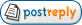Topic: OFFLINE FFTAuthor Message
angel61606

Joined: Jul 8, 2020
Posts: 55Posted: Jul 18, 2021 3:56 AM    Post subject: OFFLINE FFT Hi Jarek i have faced an interesting challenge, here is my problem: I should get an fft of my recoded signal then I calculate its median frequency , so simple, right? but then when i want to implement it, since in bioera data is read sample by sample from XDF reader , I should apply an fft which will read data sample by sample and gives output as data stream proceeds, so to measure the median frequency of the ALL of the signal, i should apply an averaging , right? but how to calculate averaging?1) should i average the outputs of fft then calculate the median frequency ? or 2)should i calculate fft then median frequency and then do averaging on the output of median frequency?I should admit both ways seem to have an incorrect result based on what i see from spectrograph !Isn't there any way to calculate FFT of the signal after the stream is read completely from xdf reader? then calculate the median frequency or any required feature?angel61606

Joined: Jul 8, 2020
Posts: 55Posted: Jul 20, 2021 1:18 AM    Post subject: I Am still waiting for an answer !